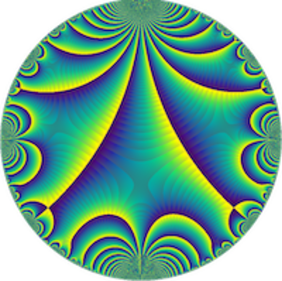# The Eisenstein ideal of weight k and ranks of Hecke algebras (Shaunak Deo, Brandeis University)

Séminaire « Arithmétique »
M2 Kampé de Fériet
Let $p$ and $\ell$ be primes such that $p > 3$ and $p \mid \ell - 1$ and $k$ be an even integer. Using deformation theory of Galois representations, we will give a necessary and sufficient condition for the $Z_p$-rank of the completion of the Hecke algebra acting on the space of cuspidal modular forms of weight $k$ and level $\Gamma_0(\ell)$ at the maximal Eisenstein ideal containing $p$ to be greater than $1$ in terms of vanishing of the cup products of certain global Galois cohomology classes.